• TOTAL函数系列 TOTAL函数系列共包含三个函数：TOTALMTD函数，TOTALQTD函数，TOTALYTD函数。 隶属于“值函数”，用途相对于DATESMTD系列函数更为直接。 用途：用来计算月初/季度初/年初迄今的累计值。 语法 语法1： ...TOTAL函数系列
TOTAL函数系列共包含三个函数：TOTALMTD函数，TOTALQTD函数，TOTALYTD函数。
隶属于“值函数”，用途相对于DATESMTD系列函数更为直接。
用途：用来计算月初/季度初/年初迄今的累计值。
语法
语法1：
DAX=
TOTALMTD(<表达式>,<日期>[,<筛选器>])

语法2：
DAX=
TOTALQTD(<表达式>,<日期>[,<筛选器>])

语法3：
DAX=
TOTALYTD(<表达式>,<日期>[,<筛选器>][,<结束日期>])

参数
表达式：返回标量的表达式，通常用来表示计算。
日期：日期列。
筛选器：（可选项）筛选条件。
结束日期：（可选项）用来定义年度结束日期，只有YEAR有，前两者没有。
返回结果
月初/季度初/年初至今的数据值累计之和。
例子
模拟数据：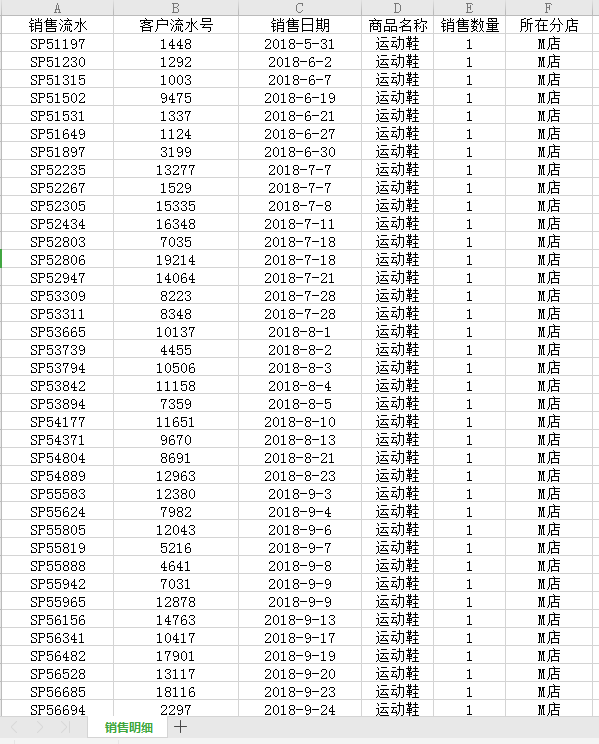这是白茶用了很多期的一份模拟数据。
例子1：
TOTALMTD例子 =
TOTALMTD ( SUM ( '销售明细'[销售数量] ), '销售明细'[销售日期])

结果：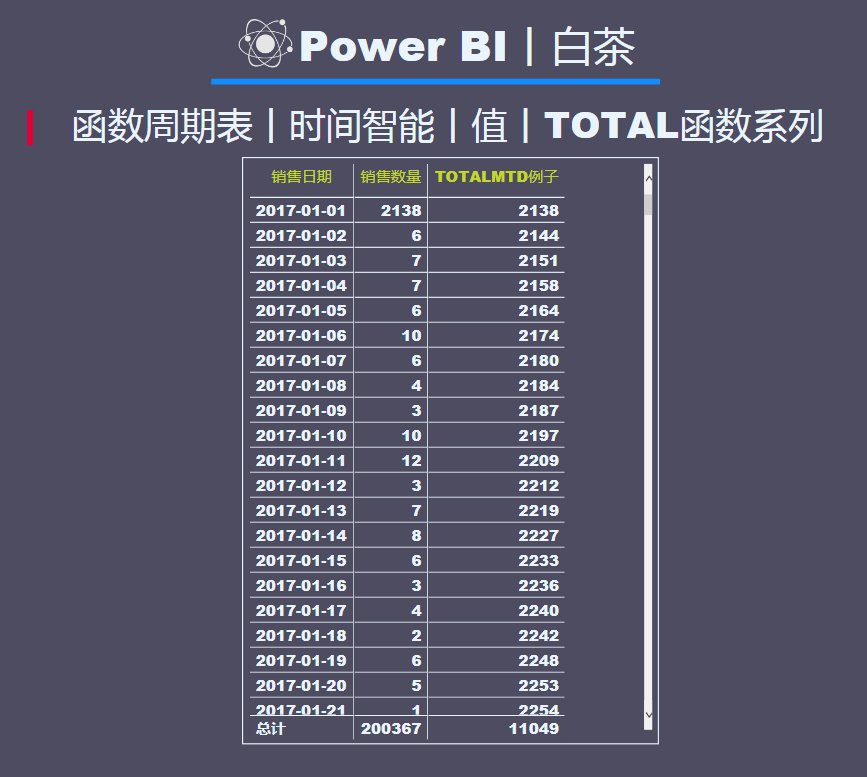按照时间维度，逐日进行累计，计算本月累计至今的数据值。
其效果等同于以下公式：
对比DATESMTD =
CALCULATE ( SUM ( '销售明细'[销售数量] ), DATESMTD ( '销售明细'[销售日期]) )

结果对比：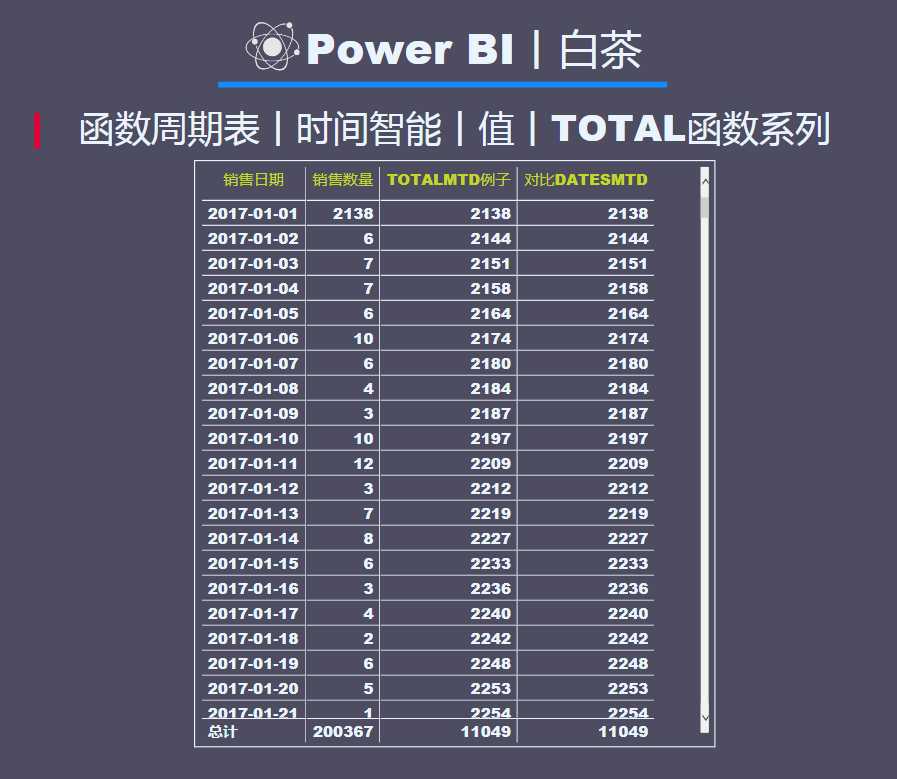可以看得出来两者的返回结果是一致的。
DATESMTD可以用来计算日期生成表，也可以与CALCULATE函数搭配求累计值。
而TOTALMTD只是单纯的计算累计值，用途更纯粹一点。
后续不再对比QTD和YTD，效果都差不多。
例子2：
TOTALQTD例子 =
TOTALQTD ( SUM ( '销售明细'[销售数量] ), '销售明细'[销售日期] )

结果：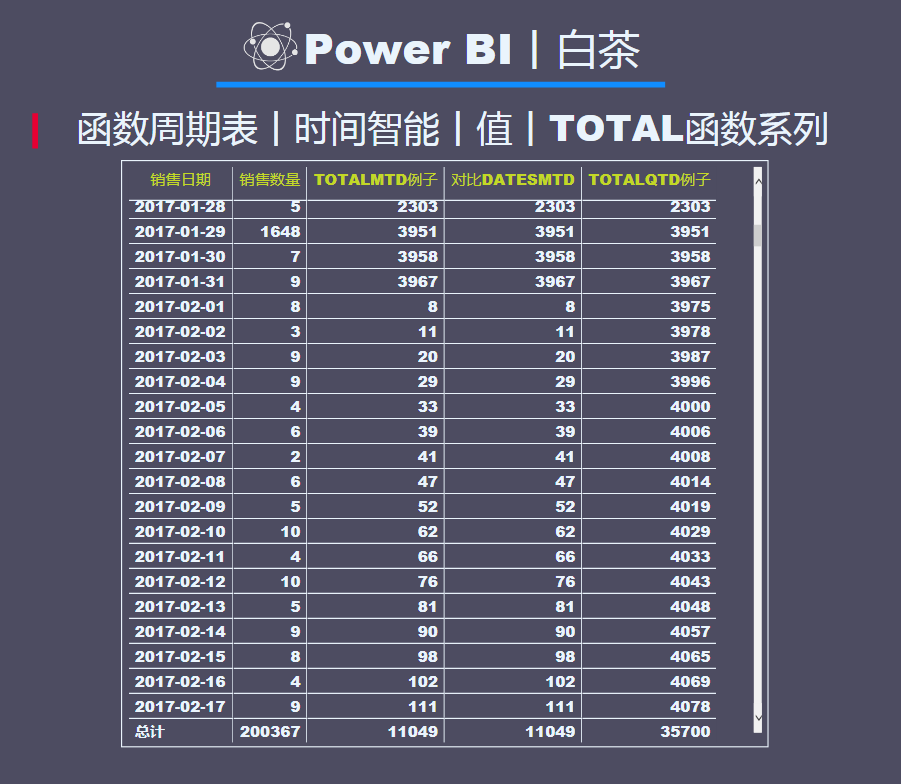逐日累计，计算本季度至今为止的累计值。
例子3：
TOTALYTD例子 =
TOTALYTD ( SUM ( '销售明细'[销售数量]), '销售明细'[销售日期] )

结果：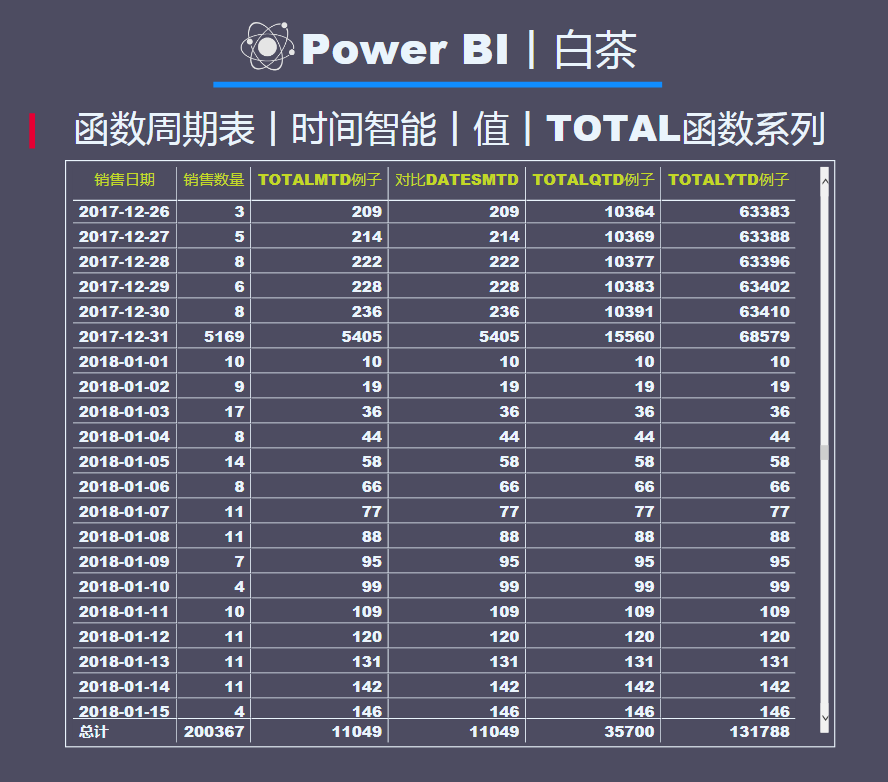逐日累计，计算本年度迄今为止的数据值累计。
到这里，时间智能函数基本上就告一段落了，对于刚开始学DAX的小伙伴来说，这一类函数作为过渡使用是再好不过的了，如果想根据具体的案例去解决具体的问题，那么还需要多多深入了解DAX哦。
小伙伴们，GET了么？
白茶会不定期的分享一些函数卡片哦。
（文件在知识星球[PowerBI丨需求圈]）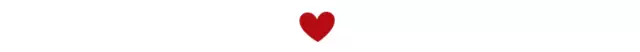这里是白茶，一个PowerBI的初学者。
下面这个知识星球是针对有实际需求的小伙伴，有需要的请加入下面的知识星球。
（这个星球里面有白茶之前所有的案例文件。）展开全文人工智能 数据分析 机器学习
• 一、对隐藏值的计算和忽略计算销量平均值公式：F4=SUBTOTAL(1,C2:C8)或者G4=SUBTOTAL(101,C2:C8)(1)小伙伴们有没有发现，图1中如果没有隐藏行时，两个公式的计算结果是相同的。也就相当于=SUM(C2:C8)/7(2)当图2中把...
一、对隐藏值的计算和忽略计算销量平均值公式：F4=SUBTOTAL(1,C2:C8)或者G4=SUBTOTAL(101,C2:C8)(1)小伙伴们有没有发现，图1中如果没有隐藏行时，两个公式的计算结果是相同的。也就相当于=SUM(C2:C8)/7(2)当图2中把序号1、2行隐藏后，G4结果发生了变化。公式F4=SUBTOTAL(1,C2:C8)中的第一个参数为1，所以它就包含隐藏的行；相当于=SUM(C2:C8)/7公式G4=SUBTOTAL(101,C2:C8)中的第一个参数为101，所以它就不包含隐藏的行。相当于=SUM(C4:C8)/5二、对筛选值的忽略统一当前值的平均值公式 =SUBTOTAL(1,C2:C8)公式=SUBTOTAL(101,C2:C8)通过筛选数据，小伙伴有没有发现无论是使用哪个公式，结果都在发生变化，而且只对当前显示的数值负责。这就得出：通过筛选数据，不管是何种类型的统计，其计算结果只对当前筛选保留的数据复制三、永远保持连续的序号公式A2=SUBTOTAL(103,$B$2:B5)，按Ctrl Enter填充隐藏或取消隐藏行，其行号都是连续的。参数103所对应的函数为：Counta。统计非空单元格的个数。当参数为1**时，忽略隐藏的行。所以公式=SUBTOTAL(103,$B$2:B5)统计的就是从B2开始到当前单元格累计非空单元格个数。四、按颜色求和公式G10==SUBTOTAL(9,C:C)当subtotal函数第一个参数为9，那么就是筛选求和。选中C列中某一个颜色的单元格，然后右击--筛选--按所选单元格的颜色进行筛选，原理：通过筛选和subtotal函数来实现按颜色求和。
展开全文• ## 函数

2018-07-23 18:21:10

函数

def add(m,n):
total=m+n
return total
函数的参数可否改变
可变传的是地址，不可变传的是数值

a=1
m=m+2
return m
add(a)

a没有改变
在python中没有函数重载，函数名相同上面那个就失效了
关键字参数
（**）是任意关键字，没有是特定关键字，必须要有这个关键字

def add(m,n,*args,**length):
res=m+n
for i in args:
res=res+i
print(res)
print(length)

kw={'age':'12'}

add(1,2,4,5,**kw)
变量作用域
函数具有自己的作用域，函数访问变量先找自己的再找外面的。
全局变量
函数对全局变量只有访问没有编辑权限

a=1
def func_out():
global a#没有global会重新声明一个a
a=2
print(a)
func_out()
print(a)

输出结果是2，2
如果没有global，输出结果是2，1
lambda函数有自己的作用域

def makeAction():
acts=[]
for i in range(5):
acts.append(lambda x,j=i:j**x)#j会拷贝i，不会共享
return acts
fs=makeAction()
print(fs(3))
结果输出：1
内置函数（系统自带的函数）

map()

arr=[2,3,5,6,9]
res01=map(lambda x:x*2+1,arr)
print(list(res01))
输出：[5, 7, 11, 13, 19]
reduce()

arr=[2,4,6]
from functools import reduce
res01=reduce(lambda x,y:str(x)+str(y),arr)
print(res01)
输出：246
过滤

arr=['张三丰','张文','张震','王洋']
res=filter(lambda s:s=='张'and len(s)==2,arr)
print(list(res))
输出：['张文', '张震']
sorted()排序

按id降序排列
sorted(students, key=lambda s: s['id'], reverse=True)

展开全文• Total variation loss常被用在损失函数里的正则项，可以起到平滑图像，去除鬼影，消除噪声的作用 TVloss的表达式如下所示： 下面是在pytorch中的代码： import torch import torch.nn as nn from torch.autograd ...
Total variation loss常被用在损失函数里的正则项，可以起到平滑图像，去除鬼影，消除噪声的作用
TVloss的表达式如下所示：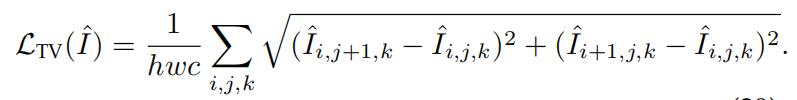下面是在pytorch中的代码：
import torch
import torch.nn as nn

class TVLoss(nn.Module):
def __init__(self,TVLoss_weight=1):
super(TVLoss,self).__init__()
self.TVLoss_weight = TVLoss_weight

def forward(self,x):
batch_size = x.size()
h_x = x.size()
w_x = x.size()
count_h =  (x.size()-1) * x.size()
count_w = x.size() * (x.size() - 1)
h_tv = torch.pow((x[:,:,1:,:]-x[:,:,:h_x-1,:]),2).sum()
w_tv = torch.pow((x[:,:,:,1:]-x[:,:,:,:w_x-1]),2).sum()
return self.TVLoss_weight*2*(h_tv/count_h+w_tv/count_w)/batch_size

def main():
x = Variable(torch.FloatTensor([[[1, 2, 3], [2, 3, 4], [3, 4, 5]], [[1, 2, 3], [2, 3, 4], [3, 4, 5]]]).view(1, 2, 3, 3),requires_grad=True)

if __name__ == '__main__':
main()



展开全文深度学习 pytorch
•Cognos 实例 占比
• 解决使用mysql统计类函数为null,而不能自定义返回值的问题 SELECT COALESCE(SUM(total),0) FROM test_tablemysql select
• Total variation loss/total variation regularization/Total variation denoising ...  总变差有点像衡量一个函数变化程度的函数。BV模型一定程度上是L1图像模型。   用在图像上，total varia...
• 查看更多： https://www.yuque.com/docs/share/922b97d2-b4e4-45bd-b567-e2616e4d1358PHP Filesystem
• 一、TOTALYTD 释义：计算当前上下文中表达式的年初至今值 语法：TOTALYTD(表达式,日期,[筛选条件],[年末日期]) 参数： 第一参数：...USD[SalesAmount_USD]),DateTime[DateKey]) 注：TOTAL系列函数不支持DirectQuery模式power
• 1. 语句i_frame_total = p_get_frame_total( opt->hin )，实现得到输入文件的总帧数，由于p_get_frame_total = get_frame_total_yuv（见Parse（）函数），所以调用函数int get_frame_total_yuv( hnd_t handle )，在...parameters file null input
• 之前遇到这样的小问题，记得我之前是将图像转换为数组的形式存储，之后处理需要转换为二维的数组，大概就是这样。这里就不还原了，我用个简单的小...首先看看报错信息： **total size of new array must be unchangepython 异常处理
• def sum(x, y): return x+y # 定义sum函数，有两个参数x,y，返回的结果是这两个值的和 def total(x, y, z): sum_of_two = sum(x, y) sum_of_three = sum(sum_of_two, z) ...#定义total函数，有3个参...
• 我在写一个条件分页查询时，使用mybatis-plus遇到一个问题， ...这里我使用了ifnull聚合函数结果reviewStatus 作为条件查询，分页查询时报错：在进入paginationInterceptor queryTotal找不到clounm...
• 函数 在JS当中，函数就是一个方法，(一个功能体)，基于函数一般都是为了实现某个功能，并且在后续的代码中我们依然想实现相同的操作 函数诞生的目的就是为了实现封装：把实现一个功能的代码封装到一个函数中，后期...js
• 匿名函数 匿名函数指一类无须定义标识符的函数或子程序。Python用lambda语法定义匿名函数，只需用表达式而无需申明。(省略了用def声明函数的标准步骤)。 #定义匿名函数： sum = lambda arg1,arg2 : arg1+arg2 #调用...
• 当类没有定义构造函数时，当对象声明时，默认构造函数调用,不用任何参数初始化 类没有构造函数，编译器假设有隐含默认的构造函数 类的对象通过简单的声明可以构造 class Example { public: int total; void ...
• 函数指针 1,如何表示指针：指针标识符 2.如何知道是函数：() 3.函数指针是专用的，格式要求很强（参数类型，个数，返回值），就像数组指针一样 #include <stdio.h> #include <stdlib.h> int ansa(int ...指针
• 用lambda关键词能创建小型匿名函数。这种函数得名于省略了用def声明函数的标准步骤。 lambda函数的语法只包含一个语句，如下： lambda [arg1 [,arg2,…argn]]:expression 如下实例： sum = lambda arg1, arg2: arg1 ...
• 实现求均值 def func2(total,count): ''' 实现求多个数据的平均值 :param total: 这个形参是指所有数据的和 :param count: 这个形参是指数据的个数 ...lambda求均值，携带参数的匿名函数 avg=l...
• 在 JS 中，函数就是一个方法，一般都是为了实现某个功能。 1. 函数的作用和创建 var total = 10; total += 10; total = total/2; total = total.toFixed(2); //=&gt; 保留小数点后面两位，toFixed 时候数字包装...
• TypeScript函数js函数定义ts函数定义 js函数定义 function hello() {} const hello1 = function () {}; const hello2 = () => {}; ts函数定义 ... * 有参数的函数 ... * 返回类型根据情况添加...const total2 = add(1,typescript
• count函数里加函数 PHP count()..."count() function" is used to get the total number of elements of an array. “ count()函数”用于获取数组元素的总数。 Syntax: 句法： count(array, [count_mode]) H...php python numpy leetcode laravel
• 普通函数 def func2(total,count): ''' 实现求多个数据的平均值 :param total: 这个形参是指所有数据的和 :param count: 这个形参是指数据的个数 :return: 均值 ''' avg = total/count return avg 匿名函数 变量名=...python
• # -*- coding: utf-8 -*- # 类函数 和 静态函数 class People(object): ... total = 0 def __init__(self, name, age): # 调用父类的初始化函数 super(People, self).__init__() # 初始化当前类python
• ---恢复内容开始--- 1、函数的定义 function 函数名字(){ 　代码块 } 2、函数调用 ...函数名字()；...function total(){ 　for(var k in arguments){ 　sum+=arguments[k]; 　} 　document...
• <p>@param $bytes : total amount in bytes of data consumed @param$rate : rate per GB, example .22 (for 22 cents) <p>@returns Total amount owed <p>Rounded to nearest cent of course. Thanks in advance...function math php
• # -*- coding:utf-8 -*- 1. 类函数 和 静态函数 调用父类的初始化函数  super(People, self).__init__() class People(object): ... total = 0 def __init__(self, name, age): # 调用父类的初...